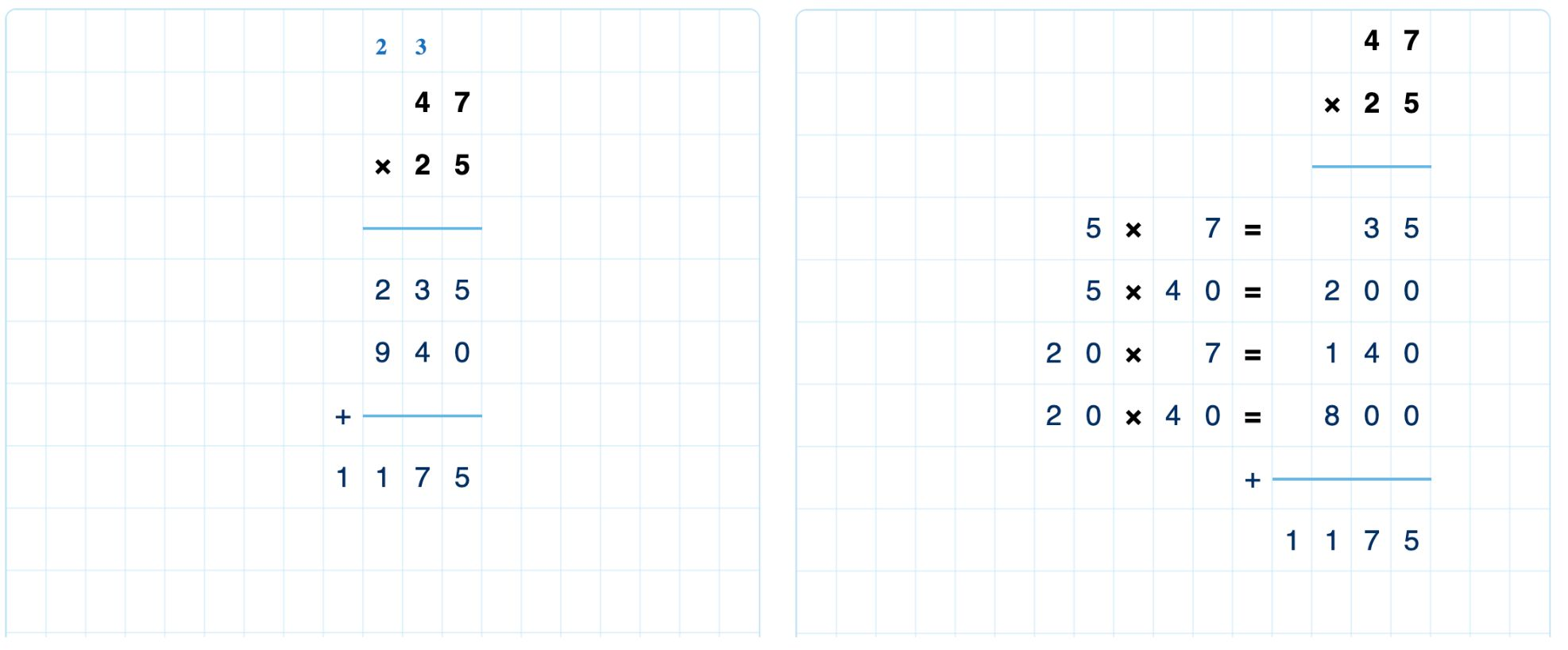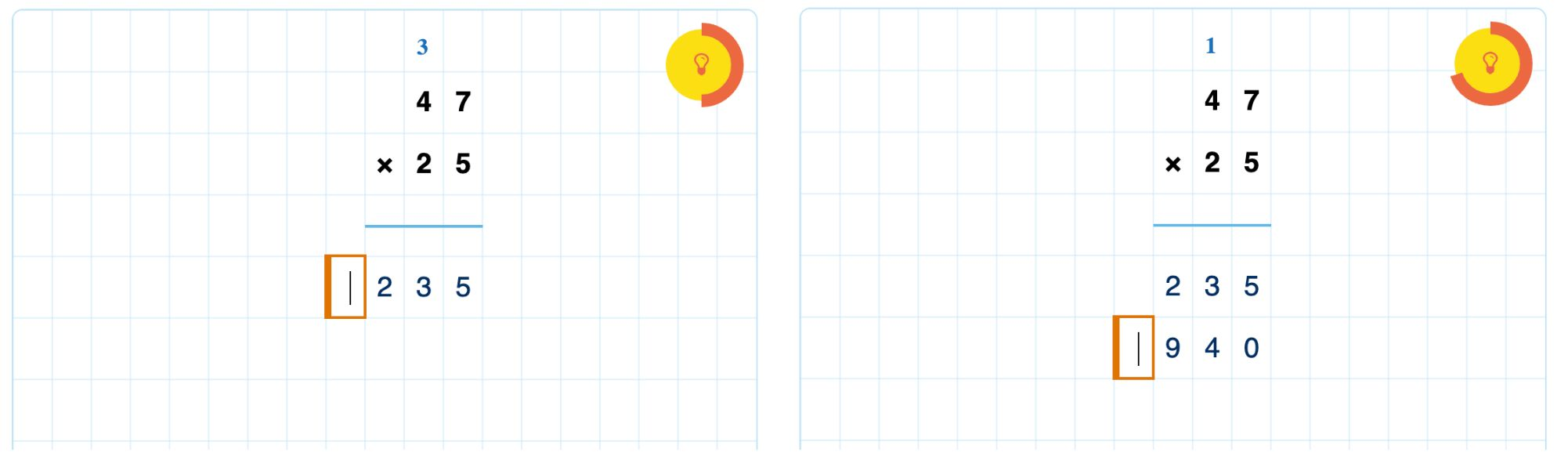# Multi-digit multiplication

## Introduction

Multi-digit multiplication shares many of the features of multi-digit addition and multi-digit subtraction, such as:

• Short and extended forms
• Options to specify elements as optional or required
• Options to specify what part of the problem is visible at the start
• Option to display a place value ribbon.
Note

Multi-digit multiplication does not support the multiplication of two decimal numbers, although one of the two factors can be decimal.Figure: Short and extended forms for multiplication.

## Regrouping

The short form of multiplication uses regrouping when the result of multiplying two digits cannot be written as a single digit. You can configure whether students must show regrouping in their solution or if this is optional.Figure: The regrouping area resets when multiplying the next place value.

Students must use the same regrouping area more than once when multiplying with a value of two or more digits. In the image above, multiplying by 5 requires a carry of 3 for the tens. When the student is ready to multiply by 20, Algebrakit clears the regrouping area automatically. Now students can carry a 1 when multiplying $7\times 2$.